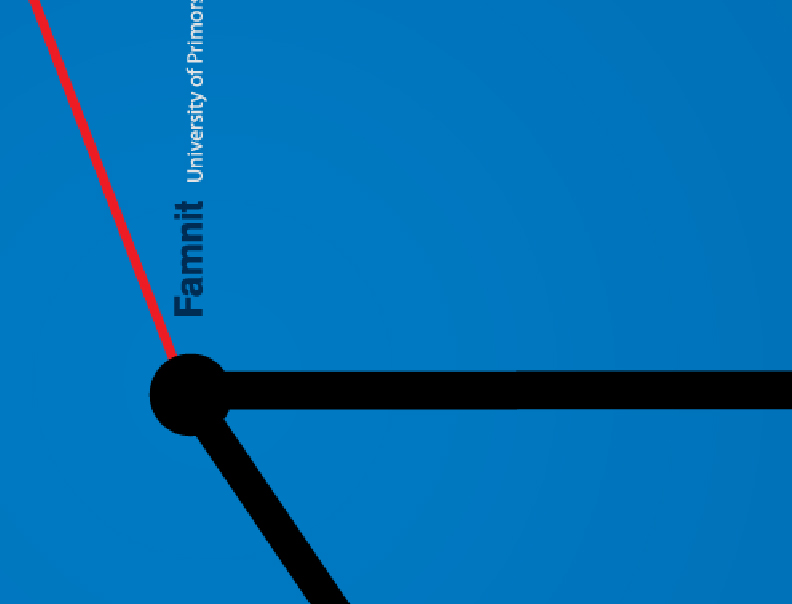Univerza na Primorskem Fakulteta za matematiko, naravoslovje in informacijske tehnologijeSI | EN

# Graph Theory Semester - Wilfried Imrich

natisni

Symmetry Breaking in Graphs

In their paper "Symmetry Breaking in Graphs" Albertson and Collins (1996) introduced the distinguishing number of a graph G It is the least cardinal d such that G has a labeling with d labels that is only preserved by the trivial automorphism. The idea is to break automorphisms of a graph efficiently by coloring the vertices with a minimum number of colors.

Their paper proved to be extremely seminal and has lead to a multitude of publications, mostly on finite graphs.

This course gives a concise presentation of selected results on finite graphs and continues with generalizations to locally finite, connected infinite graphs, and then to graphs of higher cardinality.

Many results have classical proofs, some are probabilistic, some need transfinite induction, and others depend on characterizations of classes of graphs, such as products of graphs, finite abelian vertex transitive graphs, or infinite vertex transitive graphs of polynomial growth.

In some cases algorithms are needed - and developed - to find the answer.

Much of the time will be devoted to the infinite motion conjecture by Tom Tucker, with new results and open problems pertaining to this conjecture.

No special prerequisites are required for the course, but a basic understanding of graph automorphisms will be helpful. Selected papers and class notes will be provided.

Lecturer: Wilfried Imrich, University of Leoben, Austria